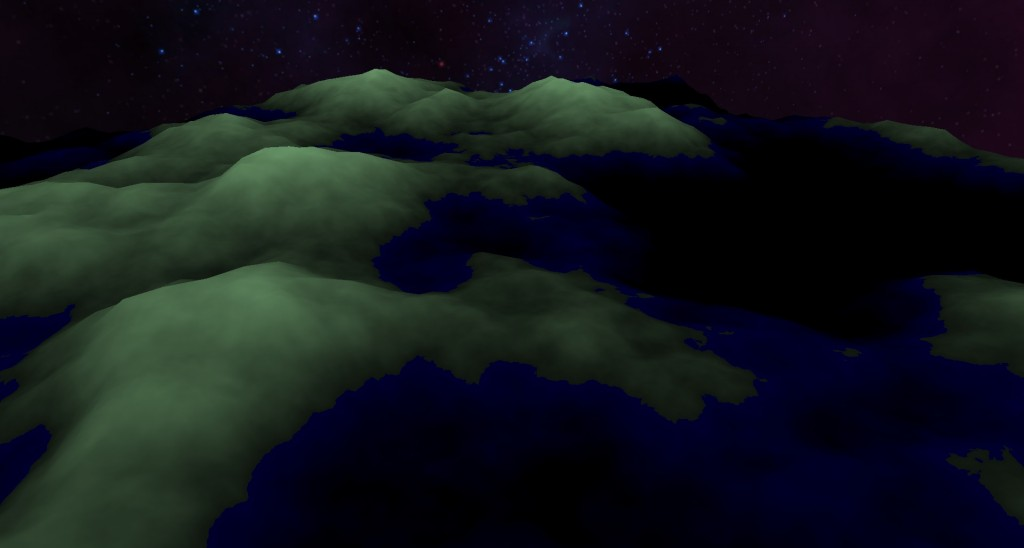# Procedural Fractal TerrainAfter doing atmospheric scattering I though that it would be an interesting challenge to make a full scale planet renderer. This is what I started with when looking to generate the terrain. The terrain height and color is determined through a height map that I generate in the vertex and fragment shaders.

I generated the height map using the 2D simplex noise implementation from webgl-noise implemented in the vertex and fragment shaders. Simplex noise is similar to Perlin noise in how it looks visually and gives a slight performance increase when using 2D and 3D noise functions. Alone, simplex noise would make for pretty boring terrain. However, if you add two or more functions of simplex noise together you start to get some more detail. This is called Fractional Brownian Motion, and is what I have used to create these fairly detailed height maps.

This what the factional Brownian motion function that I use looks like.

```float fbm(vec3 x, float initialFrequency, float lacunarity, float gain, int octaves)
{
float total = 0.0f;
float frequency = initialFrequency;
float amplitude = gain;

for (int i = 0; i < octaves; ++i)
{
total += simplexNoise(x * frequency) * amplitude;
frequency *= lacunarity;
amplitude *= gain;
}